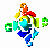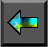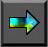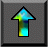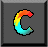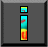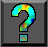Next: 12 Further Reading Up: 11 Hypercube Algorithms Previous: Exercises

# Chapter Notes

Leighton  discusses basic properties of hypercubes and describes many parallel algorithms that use a hypercube communication structure. The recursive halving vector reduction algorithm considered in Section 11.2 is described by Fox et al.  and the hybrid algorithm by van de   Geijn . Vector broadcast algorithms are described by Bertsekas and Tsitsiklis  and Johnsson and Ho . The parallel mergesort algorithm, often   called bitonic mergesort, is due to Batcher ; Akl  provides a good description. Fox et al.  describe a multicomputer implementation of this algorithm and of the variant discussed in Exercise 4. Other algorithms that are conveniently formulated in terms of a hypercube communication   structure include the fast Fourier transform [20,21,110,192,277], parallel   prefix , and various computer vision  and linear algebra computations . See also the book by Kumar et al.  and papers by Bertsekas et al. , McBryan and van der Velde , and Saad and Schultz [248,249].

The book by Kumar et al.  describes parallel versions of   several sorting algorithms, including quicksort , one of the most commonly used sorting algorithms on sequential computers. Their parallel quicksort algorithm partitions processors according to the number of elements lesser than or greater than the pivot at each step. Fox et al.  describe an alternative parallel quicksort algorithm that uses regular processor partitions. They address load imbalance by performing some preliminary processing to identify pivots that approximately bisect the input sequence. Knuth  and Aho et al.  are good references for sequential sorting algorithms. In addition to standard algorithms such as mergesort and quicksort, Knuth describes various specialized algorithms designed to exploit certain properties of the data to be sorted. Kumar et al.  explain how many of these can be adapted for parallel computers. For example, if data are highly redundant (that is, they have many identical items), we can count items on each node, then do a global sum to obtain total counts. If the input   data distribution is known, a parallel bucketsort can be used. Each processor knows the location of each bucket and sends its data to the appropriate location.

Here is a Web Tour providing access to additional information on modular programming, parallel program design, and templates.Next: 12 Further Reading Up: 11 Hypercube Algorithms Previous: Exercises